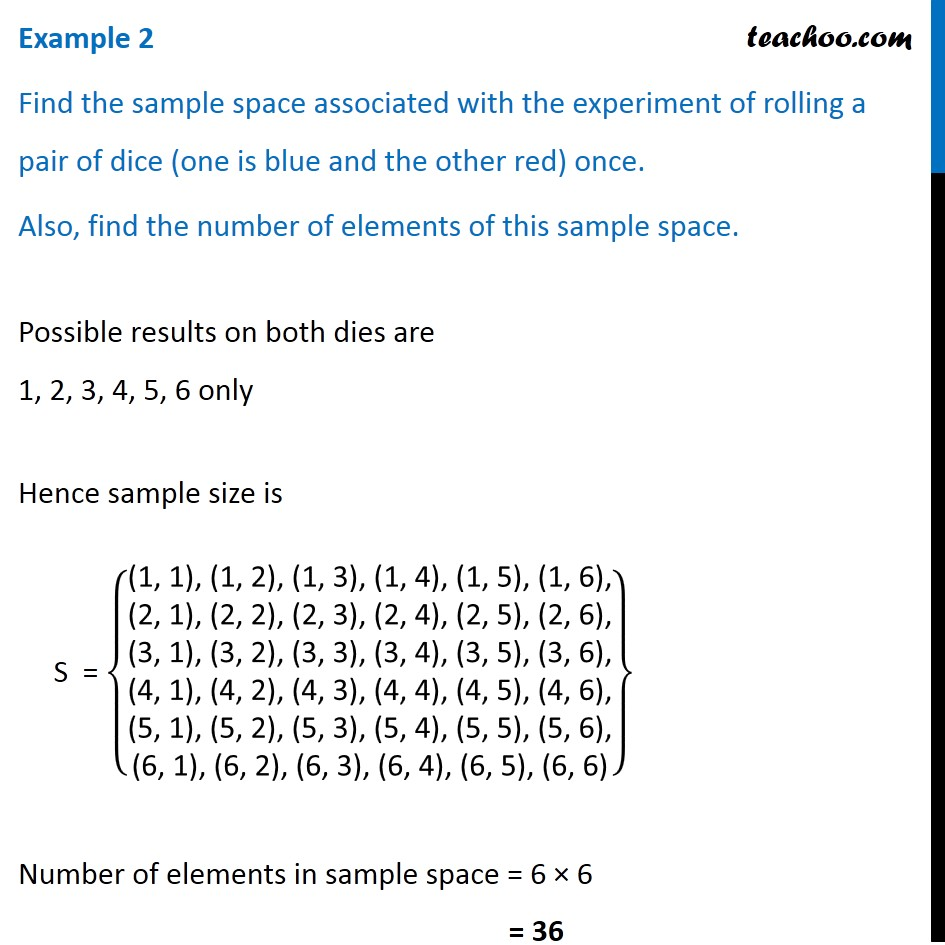Examples

Chapter 14 Class 11 Probability
Serial order wiseLearn in your speed, with individual attention - Teachoo Maths 1-on-1 Class

### Transcript

Question 2 Find the sample space associated with the experiment of rolling a pair of dice (one is blue and the other red) once. Also, find the number of elements of this sample space. Possible results on both dies are 1, 2, 3, 4, 5, 6 only Hence sample size is S = {█("(1, 1), (1, 2), (1, 3), (1, 4), (1, 5), (1, 6)," @"(2, 1), (2, 2), (2, 3), (2, 4), (2, 5), (2, 6)," @"(3, 1), (3, 2), (3, 3), (3, 4), (3, 5), (3, 6)," @"(4, 1), (4, 2), (4, 3), (4, 4), (4, 5), (4, 6)," @"(5, 1), (5, 2), (5, 3), (5, 4), (5, 5), (5, 6)," @"(6, 1), (6, 2), (6, 3), (6, 4), (6, 5), (6, 6)" )} Number of elements in sample space = 6 × 6 = 36